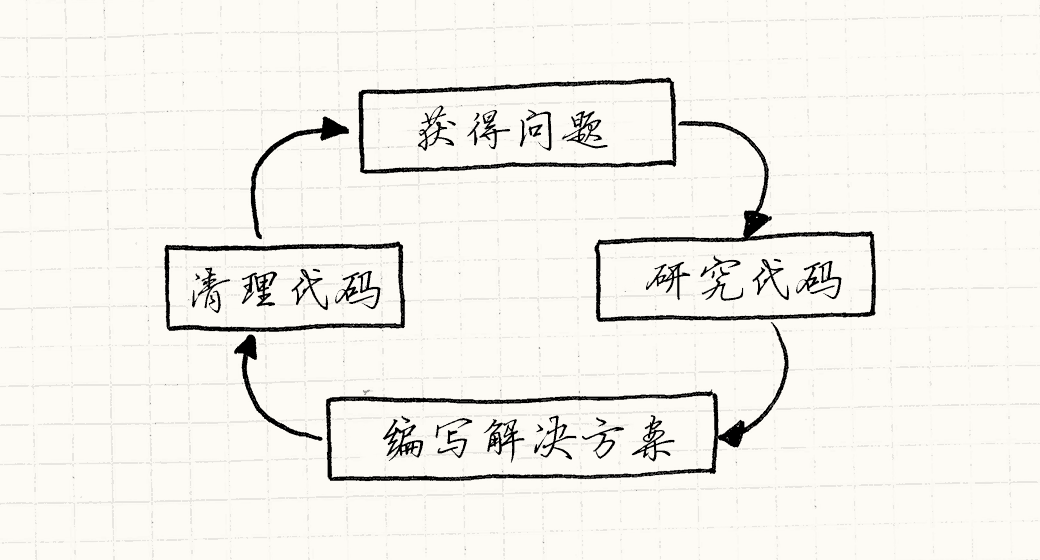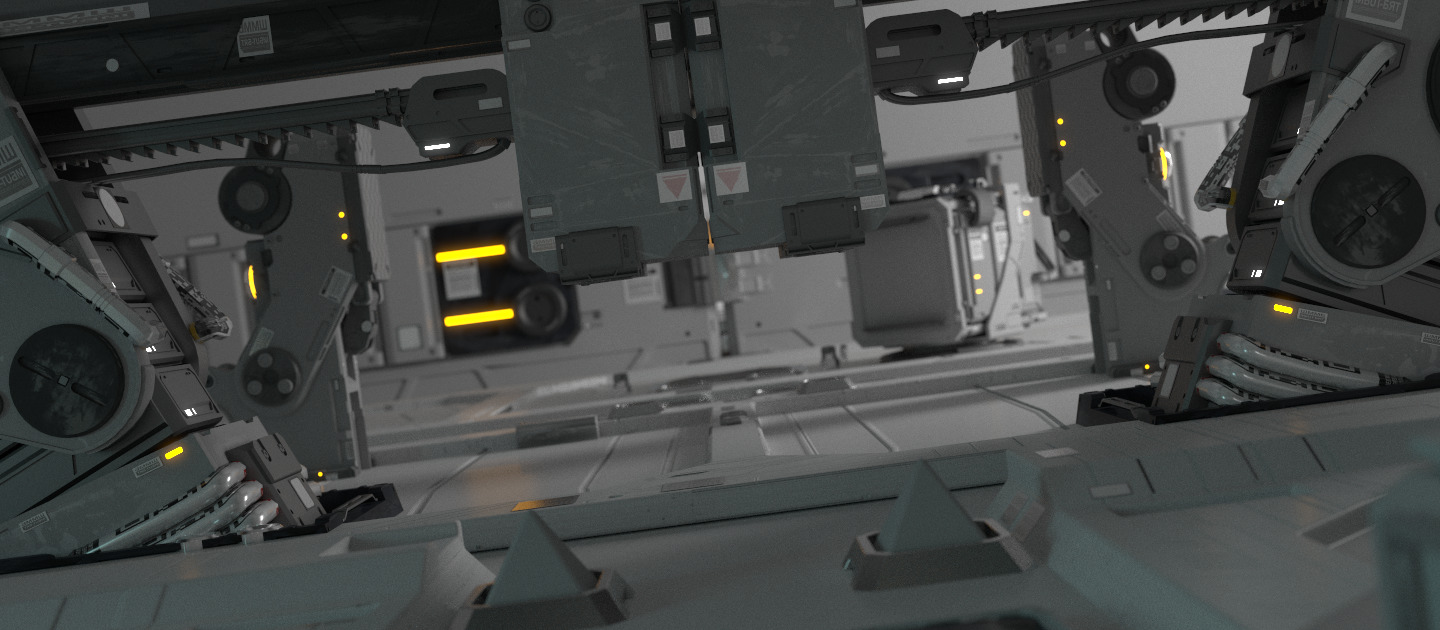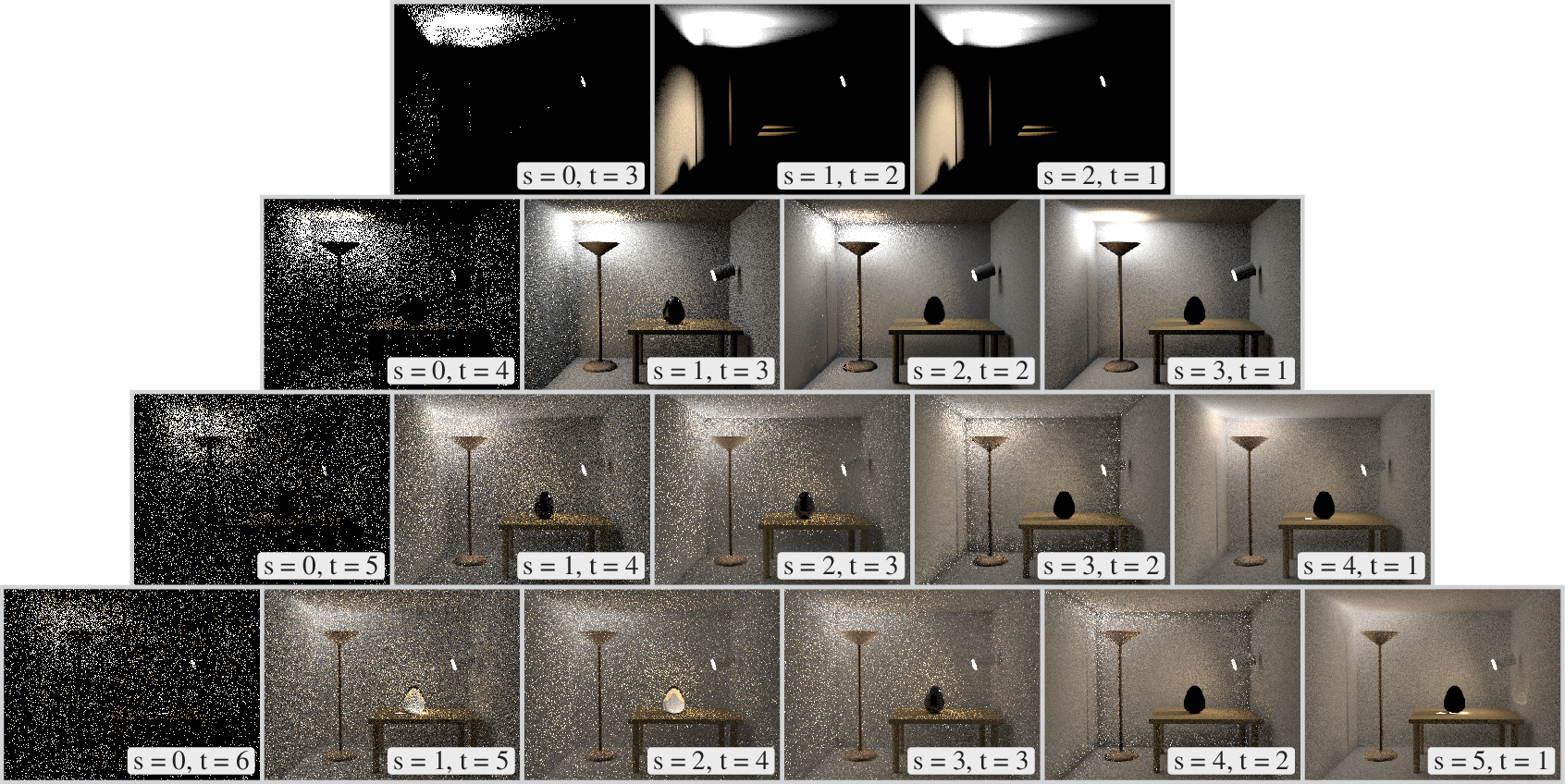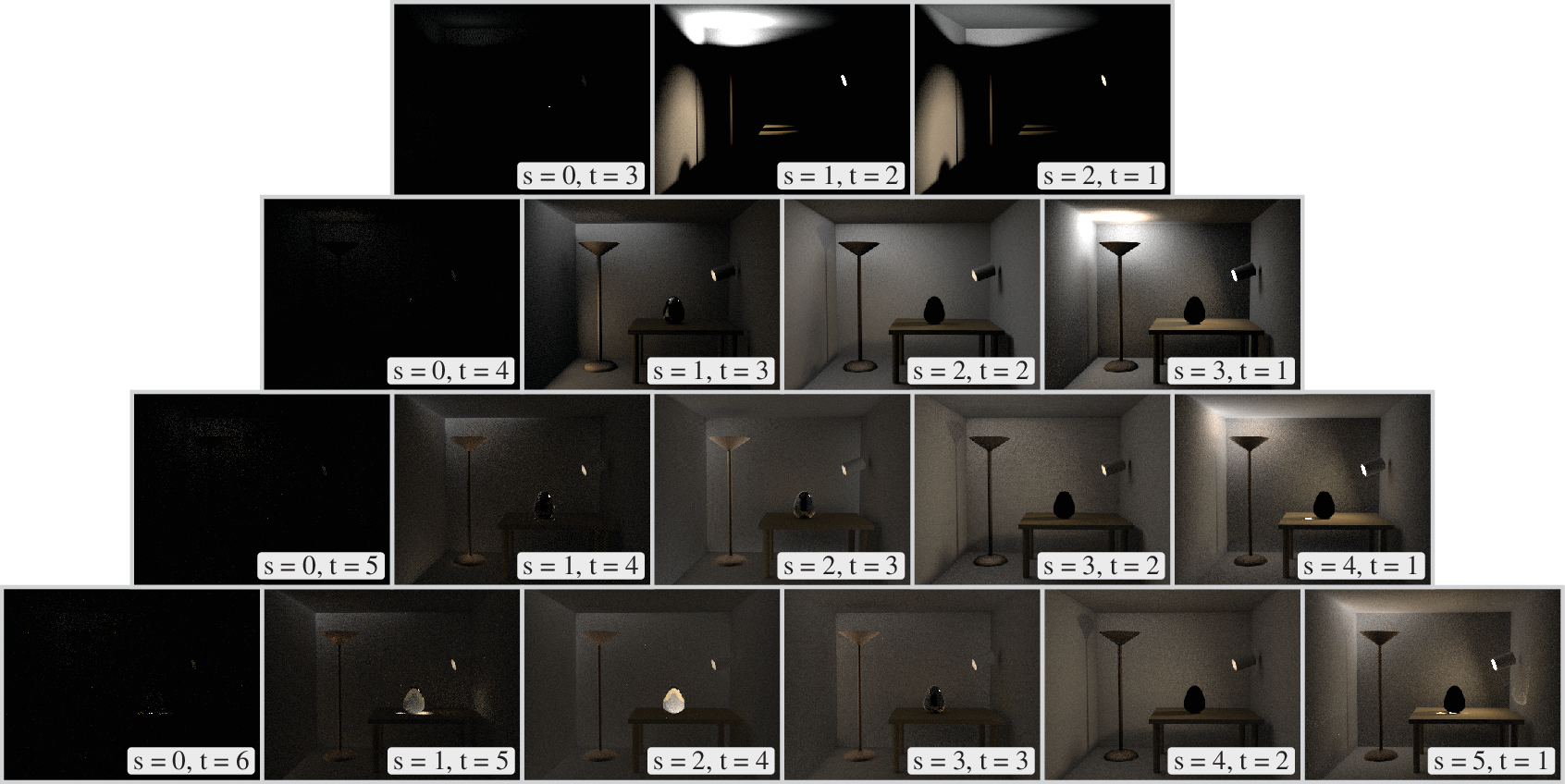Blog of Martin Z He 2022-05-07T02:08:54.221Z https://blog.apassbydreg.work/ Martin Z He Hexo GTC2022 Talk - How CUDA Programming Works https://blog.apassbydreg.work/2022/05/GTC2022-How-CUDA-Programming-Works/ 2022-05-07T02:03:12.000Z 2022-05-07T02:08:54.221Z 原始链接：How CUDA Programming Works | NVIDIA On-Demand

# 显存带宽限制1. 首先将数据从给定的行读取到感知增幅器（Sense Amplifier）中，这一操作会将行中原有的数据破坏
2. 接着从增幅器中读取给定的列，这一操作不会破坏数据
3. 如果接下来的请求来自同一行，可以重复地读取当前增幅器中的数据值，或者同时读取多列的数据
4. 当需要选择新的一行时，增幅器中的数据需要被写回显存中# 计算资源限制

## CUDA 的执行顺序CUDA 使用 SIMT 执行框架，让统一指令在多个线程之上执行。A100 的一个 SM 可以管理 64 个 warp ，它被分为 4 组不同的单元，各自拥有其对应的寄存器、指令缓存、分配器。由于 block 中的线程会共同读取数据，一个核心优化点在于让所有的线程按照线程 ID 访问相邻的内存，并尽可能地让线程数目满足读取的数据可以占满显存一行的倍数的大小

## GPU 的计算资源]]>
<p>原始链接：<a href="https://www.nvidia.com/en-us/on-demand/session/gtcspring22-s41487/?playlistId=playList-87118008-d10b-42f9-8c57-a50bbf006662
《游戏编程模式》笔记合集 | Notes Collection for Game Programming Patterns https://blog.apassbydreg.work/2022/04/GPP-Archive/ 2022-04-18T16:25:38.000Z 2022-04-18T16:35:45.493Z# 中文原文

WEB 版：https://gpp.tkchu.me/

]]>
<center><img src="https://gpp.tkchu.me/images/architecture-cycle.png" style="max-height: 40vh"></center> <p>这是一本较为入门的有趣的设计模式书籍，其中简单生动地描述了游戏中
《游戏编程模式》第五章笔记 | Notes for Game Programming Patterns Chapter 05 https://blog.apassbydreg.work/2022/04/GPP-ch05/ 2022-04-18T16:22:12.000Z 2022-04-18T16:25:10.368Z 第五章：优化模式

## 空间分区

]]>
<h1 id="第五章优化模式"><a class="markdownIt-Anchor" href="#第五章优化模式"></a> 第五章：优化模式</h1> <p>优化游戏性能是一门高深的艺术，要接触到软件的各个层面。 底层程序员掌握硬件架构的种种特质。同时，算法研究者争先恐
《游戏编程模式》第四章笔记 | Notes for Game Programming Patterns Chapter 04 https://blog.apassbydreg.work/2022/04/GPP-ch04/ 2022-04-17T16:52:30.000Z 2022-04-17T16:55:18.765Z 第四章：解耦模式

## 服务定位器

]]>
<h1 id="第四章解耦模式"><a class="markdownIt-Anchor" href="#第四章解耦模式"></a> 第四章：解耦模式</h1> <p>本章的模式有助于解耦复杂系统中的各个部分，<strong>组件模式</strong>将一个实体拆成多个，解耦不同
Dynamic Diffuse Global Illumination - GDC 2018 https://blog.apassbydreg.work/2022/04/DDGI/ 2022-04-16T04:52:53.000Z 2022-04-16T05:07:39.850Z 本文源自 GDC 2018 中 NVIDIA 的分享：https://morgan3d.github.io/articles/2019-04-01-ddgi/index.html

# Glossy GI

## 环境贴图

Glossy GI 贡献了光滑表面上可辨识的反射，从 19 世纪 70 年代起，其就被使用环境贴图来近似。随着时间的演进，它的核心算法也被视差扰动和可变表面粗糙度的方法而改进。这一类算法一般被称为环境映射、环境探针、辐照度探针、光照探针等。

## 算法1. 在只有一半的垂直分辨率的 GBuffer 上进行光线追踪，每个像素追踪一个完美反射方向，然后使用常用的延迟渲染方法对光线追踪的结果位置进行着色，次级 Glossy GI 也可以使用类似的方法完成
2. 使用 MIP map 模糊镜面反射结果的 buffer ，模糊时会使用特殊的双向滤波方法以保证其不会采样到无高光反射的黑色区域
3. 使用主表面的粗糙度和出入射光线的距离计算 MIP map 的层级，进而采样上述贴图

# 旧 Diffuse GI

## 漏光# DDGI

## 升级探针

• 对于漏光问题，将可视性信息储存在探针中
• 对于动态问题，使用异步的 GPU 光线追踪以计算低分辨率的探针，并使用一个内存一致的收集混合策略以分摊消耗，避免闪烁

## 探针放置## 数据结构## 算法

DDGI 和传统的 Glossy GI 算法类似，它主要包含了三个步骤：光线追踪、更新探针以及对可视位置进行着色。### 采样探针

DDGI 主要的性能开销在前两部分，但它们可以独立于帧率和屏幕分辨率更新，这使得其开销可以简单地分摊到应用整体上。在 RTX2080 上，这种独立性会带来约 100ms 的间接光照更新延迟，这在大部分情况下都是难以察觉的；而对于低端的显卡，间接光照会存在更多的延迟，但对于静态场景而言其质量仍然十分突出。

]]>
<p>本文源自 GDC 2018 中 NVIDIA 的分享：<a href="https://morgan3d.github.io/articles/2019-04-01-ddgi/index.html">https://morgan3d.github.io/articles/2
《GPU Gem3》第十三章笔记 - 使用后处理的体积光散射 | Notes for GPU Gems 3 Chapter 13 - Volumetric Light Scattering as a Post-Process https://blog.apassbydreg.work/2022/04/GPUGems3-ch13/ 2022-04-06T11:36:31.000Z 2022-04-06T11:48:53.652Z 本章提出了一种使用后处理方法的体积光散射效果。

# 体积散射

$L(s, \theta)=L_{0} e^{-\beta_{ex} s}+\frac{1}{\beta_{\mathrm{ex}}} E_{\text {sun }} \beta_{\mathrm{sc}}(\theta)\left(1-e^{-\beta_{ex} s}\right)$$L(s,\theta,\phi) = (1 - D(\phi))L(s,\theta)$

$L(s, \theta, \phi)=\sum_{i=0}^{n} \frac{L\left(s_{i}, \theta_{i}\right)}{n}$

$L(s, \theta, \phi)=\text { exposure } \times \sum_{i=0}^{n} \text { decay }{ }^{i} \times \text { weight } \times \frac{L\left(s_{i}, \theta_{i}\right)}{n}$

# 屏幕空间遮蔽方法

## 遮蔽 Pre-Pass 方法# 拓展

]]>
<p>本章提出了一种使用后处理方法的体积光散射效果。</p> <h1 id="缝隙光"><a class="markdownIt-Anchor" href="#缝隙光"></a> 缝隙光</h1> <p>当空气中具有了足够浓度得到气溶胶后，遮光物会投影下体积阴影，看起来像是有光线
《游戏编程模式》第三章笔记 | Notes for Game Programming Patterns Chapter 03 https://blog.apassbydreg.work/2022/03/GPP-ch03/ 2022-03-19T16:31:26.000Z 2022-03-19T16:34:37.980Z 第三章：行为模式

## 类型对象

]]>
<h1 id="第三章行为模式"><a class="markdownIt-Anchor" href="#第三章行为模式"></a> 第三章：行为模式</h1> <p>本章的模式有助于快速定义和完善大量的行为。 <strong>类型对象</strong>定义行为的类别而无需完成真
《GPU Gem3》第十二章笔记 - AO | Notes for GPU Gems 3 Chapter 12 - High-Quality Ambient Occlusion https://blog.apassbydreg.work/2022/03/GPUGems3-ch12/ 2022-03-16T12:47:02.000Z 2022-03-16T16:18:30.507Z 早期算法# 一种鲁棒的算法

## 平滑不连续位置## 去除挤压并增加细节

$F_{pA} = {1 \over 2\pi}\sum_i n\cdot{e_i \times v_i \over |e_i \times v_i|}$# 结果对比

## 渲染结果## 性能表现

n_trianglesn_fragmentsn_disks
bunny69,451381,046138,901
car29,304395,61358,607# 注意事项

## 强制收敛## 可调参数

### 距离衰减# Future Work]]>
<h1 id="早期算法"><a class="markdownIt-Anchor" href="#早期算法"></a> 早期算法</h1> <p>最开始的 AO 技术在多边形的顶点上使用 disk 近似相邻的多边形，接着将顶点周围的各个 disk 贡献的阴影累积到顶点上以近似
《游戏编程模式》第二章笔记 | Notes for Game Programming Patterns Chapter 02 https://blog.apassbydreg.work/2022/03/GPP-ch02/ 2022-03-15T12:46:55.000Z 2022-03-16T16:19:57.818Z 第二章：序列模式

## 更新方法

]]>
<h1 id="第二章序列模式"><a class="markdownIt-Anchor" href="#第二章序列模式"></a> 第二章：序列模式</h1> <p>大多数游戏世界都有的特性是时间——虚构世界以其特定的节奏运行。 作为世界的架构师，我们必须发明时间，制造推动游戏
《GPU Gem3》第十一章笔记 - Robust Shadow Volumes | Notes for GPU Gems 3 Chapter 11 - Efficient and Robust Shadow Volumes Using Hierarchical Occlusion Culling and Geometry Shaders https://blog.apassbydreg.work/2022/03/GPUGems3-ch11/ 2022-03-10T12:46:38.000Z 2022-03-16T16:24:35.497Z Shadow Map 和 Shadow Volume 是两种主流的阴影渲染方法。SM 可以很容易地用于实现软阴影，但会遇到走样的问题，SV 则可以以像素级别的精度渲染阴影，但无法很好地处理理想点光源和平行光以外的光源。

SV 的主要思想如下图所示，任何处在绿色区域的受光体均不应该被照亮，因此可以以不被照亮的形式渲染所有 SV 中的几何体，SV 外的几何体则需要计算光照。对于一根从视点出射的光线，每次当其进入 SV 时减一，离开时则加一，这样可以有效地找到 SV 内的像素。## Z-Pass 和 Z-Fail

1. 重置模板缓存为零
2. 将 SV 的边界渲染到模板缓冲中，对于正向的几何体加一，对反向的几何体减一

## 生成 Volume

### 基本步骤

1. 渲染正面（即物体本身面对光源的面片）
2. 渲染后面（即 SV 的远处面）
3. 渲染投影物的拉伸剪影（即 SV 的侧面）

# 来自 Mental Images 的实现

## 稳定的低质量 Mesh 阴影

### 一种修改过的阴影体生成法1. 挤压没有相邻几何体的受光的边缘，这使得单面的物体同样可以投影
2. 对每个 SV 边界的边缘投影两次

## 使用 GS 动态生成 SV## 使用层次遮挡剔除（Hierarchical Occlusion Culling）提高效率]]>
《GPU Gem3》第十章笔记 - PSSM | Notes for GPU Gems 3 Chapter 10 - Parallel-Split Shadow Maps on Programmable GPUs https://blog.apassbydreg.work/2022/03/GPUGems3-ch10/ 2022-03-09T13:25:47.000Z 2022-04-06T11:45:02.290Z 在这一技术中，视椎体被按照深度被平行于视平面的平面分割为多个深度层，每个深度层都会独立生成各自的 shadow map 。这一技术主要是为了提升 shadow map 在 view space 的采样频率，并与深度带来的采样频率变化所匹配。

PSSM 中的两个核心问题是：

• 如何选择分割的位置
• 如何平衡多pass和渲染阴影带来的性能损失

# 算法描述

PSSM 算法主要分为四步：

1. 使用切割平面将视椎体分割为 m 份
2. 为这些部分计算光源的 VP 矩阵
3. 为这些部分以相同的分辨率 res 生成 PSSM
4. 将阴影应用到场景中

## 第一步：分割视椎体SM 上的单位纹素 ds 投影到视平面上的面积为 dp ，可以定义走样的误差：

$error = {\mathrm{d}p \over \mathrm{d}s} = {1 \over \tan\phi}\cdot{\mathrm{d}z \over z\mathrm{d}s}\cdot{\cos\phi\over\cos\theta}$

### Practical Split Scheme

$C_i = \lambda C_i^{\log} + (1 - \lambda)C_i^{\mathrm{uni}}$$s(z)=\int_{0}^{s} d s=\frac{1}{\rho} \int_{n}^{z} \frac{1}{z} d z=\frac{1}{\rho} \ln \left(\frac{z}{n}\right)$

$s_{i}=s\left(C_{i}^{\log }\right)=\frac{1}{\ln (f / n)} \ln \left(\frac{C_{i}^{\log }}{n}\right) = {i \over m}\\C_i^{\mathrm{log}} = n\left({f \over n}\right)^{i / m}$

$C_i^{\mathrm{uni}} = n + (f - n) {i \over m}$

## 第二步：计算光源的变换矩阵

1. 和场景无关的方法直接取完整包含分割块的部分作为 VP 矩阵2. 通过引入场景信息，可以只包含可能向视椎体中投影的所有物体即可## 第三、四步：生成 PSSM 并合成阴影

1. 多 pass 方法：这种方法是最通用的，但也由于没有利用硬件的加速从而效率较低
2. 利用 DX9 级别的硬件进行部分加速：这一方法可以去除生成阴影时需要的多 pass
3. 利用 DX10 级别的硬件进行完全加速：利用 geometry shader 的 cloning 和 instancing 进一步加速

# 基于硬件的实现方法## 准备阶段

### 使用 GS 的 Cloning 技术### 使用 Instancing# 更多的优化

• 滤波技术：使用 PCF，VSM 等方法对 SM 进行滤波以减少走样
• 打包材质：在材质采样器数量较少时，可以将不同的 SM 打包到一个材质内
• 结合不同的算法使用：一种有趣的分割算法的用途是在不同的分割内使用不同的 SM 方法，如 PSM，LiSPSM，TSM 等
• 可变的材质分辨率：在不同的分割内使用不同的分辨率以减少材质生成和储存的花费
• 在线性空间处理深度
]]>
<p>在这一技术中，视椎体被按照深度被平行于视平面的平面分割为多个深度层，每个深度层都会独立生成各自的 shadow map 。这一技术主要是为了提升 shadow map 在 view space 的采样频率，并与深度带来的采样频率变化所匹配。</p> <p>PSSM 中的两个
《游戏编程模式》第一章笔记 | Notes for Game Programming Patterns Chapter 01 https://blog.apassbydreg.work/2022/03/GPP-ch01/ 2022-03-01T14:56:12.000Z 2022-03-01T15:03:40.116Z 第一章：重访设计模式

## 状态模式

]]>
<h1 id="第一章重访设计模式"><a class="markdownIt-Anchor" href="#第一章重访设计模式"></a> 第一章：重访设计模式</h1> <p>这一部分重新审视了《设计模式：可复用面向对象软件的基础》一书，从部分设计模式中提取出有趣的部分进行讲
《Physically Based Rendering 3rd ED》笔记合集 | Notes Collection for Physically Based Rendering 3rd ED https://blog.apassbydreg.work/2022/02/PBRT-Archive/ 2022-02-18T10:46:37.000Z 2022-02-18T13:42:35.636Z# 相关资源

]]>
<p><img src="https://pbr-book.org/3ed-2018/openers/measure-one180.jpg" alt="https://pbr-book.org/3ed-2018/openers/measure-one180.jpg" /></p>
PBRT 第十七章笔记 | Notes for PBRT Chapter 17 - Retrospective and The Future https://blog.apassbydreg.work/2022/02/PBRT-ch17/ 2022-02-18T10:43:39.000Z 2022-02-18T10:47:52.204Z 17.1 Design Retrospective

PBRT 在设计上的一个基础的假设在于：支持复杂和多样的场景、形状、材质、光源和采样算法具有非常高的重要性，且为了得到这样的效果，付出计算开销是值得的。这种假设的一个后果在于 PBRT 在简单场景的渲染效率上表现不佳。

## 17.1.1 Triangles Only

• 渲染器可以依赖于三角形顶点可以直接转换到世界坐标下，而无需进行到物体坐标系的转换
• 加速结构可以更加的专一化，让其中的节点直接储存对应的三角形而非整个几何体，这可以提高内存局部性，并避免多层虚函数的调用
• displacement mapping 在这种情况下更容易实现

## 17.1.4 Specialized Compilation

2010 年提出的 OptiX 光线追踪渲染系统具有非常有趣的设计，它在内置的功能基础上可以被用户提供的代码进行拓展。很多近年的渲染器都允许经过插件等方法提供一定的用户拓展性，但 OptiX 特别在于它可以构建于一个运行时编译系统之上。由于编译器拥有对于整个系统的信息，产生的自定义渲染器可以快速且自动地专一应用于一系列内容中。

# 17.2 Alternative Hardware Architectures

CPU 一种被设计为一个注重串行性能的处理器，随着可编程图像处理器的出现，流处理器在现代计算系统中正在变得越来越重要。这类处理器并不特别关注单线程的效率，而是注重多个并行线程的表现。这种特性让流处理器对缓存、分支预测的需求变低，从而可以在同样的芯片面积上提供更多的 ALU 用于计算。

SIMD 是流处理器的关键机制，现代 CPU 和 GPU 都有在其核心内拥有 SIMD 向量单元，而其中的核心和并行数量也正在随着时间迅速增长。

## 17.2.2 Packet Tracing

Packet Tracing 在 CPU 上通常被显式地实现。相交函数会接受光线数量作为输入。与之相对的，对于流处理器的向量化操作通常是隐式的。代表通常被写做和接受单一数据输入，并被底层的编译器和硬件并行执行。

Reshetov 等人在 2005 年发表的论文中拓展了 Packet Tracing 方法，其中使用了锥体完成加速结构的遍历以提供高效的光线追踪。而后被 Reshetov 和 Benthin 与 Wald 等人进一步发展到剔除和阴影光线上。

Embree System 是一个同时支持高效单射线遍历和 Packet Tracing 的开源渲染系统，于 2014 年被 Wald 等人在论文中提出。

# 17.3 Conclusion

]]>
<h1 id="171-design-retrospective"><a class="markdownIt-Anchor" href="#171-design-retrospective"></a> 17.1 Design Retrospective</h1> <p>PBRT
PBRT 第十六章笔记 | Notes for PBRT Chapter 16 - Light Transport III > Bidirectional Methods https://blog.apassbydreg.work/2022/02/PBRT-ch16/ 2022-02-15T09:29:46.000Z 2022-02-18T10:46:54.490Z 在之前所提到的渲染方法中，均只考虑了从摄像机出发的光线，并仅在路径的终点处尝试寻找光源。本章则要讨论从光源和摄像机双向同时采样路径的方法。这些采样方法可以比单向的算法更高效地收敛。

# 16.1 The Path-Space Measurement Equation

measurement equation（测量方程）描述了一种由积分一系列带有辐照度的光线得到的抽象的度量。例如在最简单的，最简化的成像模型中计算图像中的像素 $j$ 的情况下，我们可以把该像素的值写成在图像平面上积分的如下形式：

\begin{aligned}I_{j} &=\int_{A_{\text {film }}} \int_{\mathrm{S}^{2}} W_{\mathrm{e}}^{(j)}\left(\mathrm{p}_{\text {film }}, \omega\right) L_{\mathrm{i}}\left(\mathrm{p}_{\text {film }}, \omega\right)|\cos \theta| \mathrm{d} \omega \mathrm{d} A\left(\mathrm{p}_{\text {film }}\right) \\&=\int_{A_{\text {film }}} \int_{A} W_{\mathrm{e}}^{(j)}\left(\mathrm{p}_{0} \rightarrow \mathrm{p}_{1}\right) L\left(\mathrm{p}_{1} \rightarrow \mathrm{p}_{0}\right) G\left(\mathrm{p}_{0} \leftrightarrow \mathrm{p}_{1}\right) \mathrm{d} A\left(\mathrm{p}_{1}\right) \mathrm{d} A\left(\mathrm{p}_{0}\right)\end{aligned}

$W_{\mathrm{e}}^{(j)}\left(\mathrm{p}_{0} \rightarrow \mathrm{p}_{1}\right)=f_{j}\left(\mathrm{p}_{0}\right) \delta\left(t\left(\mathrm{p}_{0}, \omega_{\text {camera }}\left(\mathrm{p}_{1}\right)\right)-\mathrm{p}_{1}\right)$

\begin{aligned}I_{j} &=\int_{A_{\mathrm{film}}} &\int_{A} W_{\mathrm{e}}^{(j)}\left(\mathrm{p}_{0} \rightarrow \mathrm{p}_{1}\right) L\left(\mathrm{p}_{1} \rightarrow \mathrm{p}_{0}\right) G\left(\mathrm{p}_{0} \leftrightarrow \mathrm{p}_{1}\right) \mathrm{d} A\left(\mathrm{p}_{1}\right) \mathrm{d} A\left(\mathrm{p}_{0}\right) \\&=\sum_{i} &\int_{A} \int_{A} W_{\mathrm{e}}^{(j)}\left(\mathrm{p}_{0} \rightarrow \mathrm{p}_{1}\right) P\left(\overline{\mathrm{p}}_{i}\right) G\left(\mathrm{p}_{0} \leftrightarrow \mathrm{p}_{1}\right) \mathrm{d} A\left(\mathrm{p}_{1}\right) \mathrm{d} A\left(\mathrm{p}_{0}\right) \\&=\sum_{i} &\underbrace{\int_{A} \cdots \int_{A}}_{i+1 \text { times }} W_{\mathrm{e}}^{(j)}\left(\mathrm{p}_{0} \rightarrow \mathrm{p}_{1}\right) T\left(\overline{\mathrm{p}}_{i}\right) L_{\mathrm{e}}\left(\mathrm{p}_{\mathrm{i}+1} \rightarrow \mathrm{p}_{\mathrm{i}}\right) G\left(\mathrm{p}_{0} \leftrightarrow \mathrm{p}_{1}\right) \\&&\mathrm{d} A\left(\mathrm{p}_{\mathrm{i}+1}\right) \cdots \mathrm{d} A\left(\mathrm{p}_{0}\right) \end{aligned}

## 16.1.1 采样相机光线

1. 计算光线和摄像机朝向的夹角，检查光线是否和摄像机朝向一致
2. 计算光栅位置，并按需写入参数指定的地址
3. 对于采样区域外的位置，返回零值
4. 计算重要性的函数值

PerspectiveCamera 类在定义上为了防止出现物理中的小孔成像的暗角问题，在 PDF 设计上加入了光线方向的影响。对于面积为 $A$ 的成像平面，其 PDF 定义为：

$p(\omega) = {d^2 \over A\cos^3\theta} ,\ \mathrm{if \ \omega \ is \ within \ frustum, \ else \ 0}$

$p(\omega) = {d^2 \over A\pi r^2\cos^4\theta} ,\ \mathrm{if \ \omega \ is \ within \ frustum, \ else \ 0}$

## 16.1.2 采样光源光线

### 方向光## 16.1.3 非对称散射

$T\left(\overline{\mathrm{p}}_{n}\right)=\prod_{i=1}^{n-1} f\left(\mathrm{p}_{i+1} \rightarrow \mathrm{p}_{i} \rightarrow \mathrm{p}_{i-1}\right) G\left(\mathrm{p}_{i+1} \leftrightarrow \mathrm{p}_{i}\right)$

### 折射带来的非对称性

$L_i = \frac{\eta_i^2}{\eta_t^2}L_t$

$f^{*}\left(\mathrm{p}, \omega_{\mathrm{o}}, \omega_{\mathrm{i}}\right)=f\left(\mathrm{p}, \omega_{\mathrm{i}}, \omega_{\mathrm{o}}\right)=\frac{\eta_{\mathrm{t}}^{2}}{\eta_{\mathrm{i}}^{2}} f\left(\mathrm{p}, \omega_{\mathrm{o}}, \omega_{\mathrm{i}}\right)$

### 着色法线带来的非对称性

$L_{\mathrm{o}}\left(\mathrm{p}, \omega_{\mathrm{o}}\right)=L_{\mathrm{e}}\left(\mathrm{p}, \omega_{\mathrm{o}}\right)+\int_{\mathrm{S}^{2}} f\left(\mathrm{p}, \omega_{\mathrm{o}}, \omega_{\mathrm{i}}\right) L_{\mathrm{i}}\left(\mathrm{p}, \omega_{\mathrm{i}}\right)\left|\mathbf{n}_{\mathrm{g}} \cdot \omega_{\mathrm{i}}\right| \mathrm{d} \omega_{\mathrm{i}}$

$f_{shade}(p, \omega_o, \omega_i) = {|\mathbf{n}_s \cdot \omega_i| \over |\mathbf{n}_g \cdot \omega_i|} f(p, \omega_o, \omega_i)\\f_{shade}^*(p, \omega_o, \omega_i) = {|\mathbf{n}_s \cdot \omega_o| \over |\mathbf{n}_g \cdot \omega_o|} f^*(p, \omega_o, \omega_i)$

$f^*(p, \omega_o, \omega_i) = {|\mathbf{n}_s \cdot \omega_o||\mathbf{n}_g \cdot \omega_i| \over |\mathbf{n}_g \cdot \omega_o||\mathbf{n}_s \cdot \omega_i|}f(p, \omega_o, \omega_i), \mathrm{while \ transporting \ importance}$

# 16.2 Stochastic Progressive Photon Mapping (SPPM)

## 16.2.1 理论基础$E\left[\frac{1}{N} \sum_{j=1}^{N} \beta_{j} W_{\mathrm{e}}\left(\mathrm{p}_{\mathrm{j}}, \omega_{\mathrm{j}}\right)\right]=\int_{A} \int_{\mathrm{S}^{2}} W_{\mathrm{e}}(\mathrm{p}, \omega) L_{\mathrm{i}}(\mathrm{p}, \omega)|\cos \theta| \mathrm{d} A \mathrm{~d} \omega$

$E\left[\frac{1}{N} \sum_{i=1}^{N} W_{\mathrm{e}}\left(\mathrm{p}_{\mathrm{i}, 0} \rightarrow \mathrm{p}_{\mathrm{i}, 1}\right)\left\{\frac{L\left(\mathrm{p}_{\mathrm{i}, 1} \rightarrow \mathrm{p}_{\mathrm{i}, 0}\right) G\left(\mathrm{p}_{\mathrm{i}, 0} \leftrightarrow \mathrm{p}_{\mathrm{i}, 1}\right)}{p\left(\mathrm{p}_{\mathrm{i}, 0}\right) p\left(\mathrm{p}_{\mathrm{i}, 1}\right)}\right\}\right]$

$\beta_{i, j}=\frac{L_{\mathrm{e}}\left(\mathrm{p}_{\mathrm{i}, \mathrm{n}_{\mathrm{i}}} \rightarrow \mathrm{p}_{\mathrm{i}, \mathrm{n}_{\mathrm{i}}-1}\right)}{p\left(\mathrm{p}_{\mathrm{i}, \mathrm{n}_{\mathrm{i}}}\right)} \prod_{j=1}^{n_{i}-1} \frac{1}{1-q_{i, j}} \frac{f\left(\mathrm{p}_{\mathrm{i}, \mathrm{j}+1} \rightarrow \mathrm{p}_{\mathrm{i}, \mathrm{j}} \rightarrow \mathrm{p}_{\mathrm{i}, \mathrm{j}-1}\right) G\left(\mathrm{p}_{\mathrm{i}, \mathrm{j}+1} \leftrightarrow \mathrm{p}_{\mathrm{i}, \mathrm{j}}\right)}{p\left(\mathrm{p}_{\mathrm{i}, \mathrm{j}}\right)}$

## 16.2.2 光子映射（Photon Mapping）

\begin{aligned}\int_{\mathrm{S}^{2}} & L_{\mathrm{i}}\left(\mathrm{p}, \omega_{\mathrm{i}}\right) f\left(\mathrm{p}, \omega_{\mathrm{o}}, \omega_{\mathrm{i}}\right)\left|\cos \theta_{\mathrm{i}}\right| \mathrm{d} \omega_{\mathrm{i}} \\&=\int_{A} \int_{\mathrm{S}^{2}} \delta\left(\mathrm{p}-\mathrm{p}^{\prime}\right) L_{\mathrm{i}}\left(\mathrm{p}^{\prime}, \omega_{\mathrm{i}}\right) f\left(\mathrm{p}^{\prime}, \omega_{0}, \omega_{\mathrm{i}}\right)\left|\cos \theta_{\mathrm{i}}\right| \mathrm{d} \omega_{\mathrm{i}} \mathrm{d} A\left(\mathrm{p}^{\prime}\right)\end{aligned}

$W_e(p', \omega) = \delta(p' - p)f(p, \omega_o, \omega)$$\hat{p}(x) = {1 \over Nh}\sum_{i=1}^N k({x - x_i \over h})$$\hat{p}(x) = {1 \over Nd_N(x)}\sum_{i=1}^N k({x - x_i \over d_N(x)})$

$\hat{p}(x) = {1 \over N(d_N(x))^d}\sum_{i=1}^N k({x - x_i \over d_N(x)})$

$L_{\mathrm{o}}\left(\mathrm{p}, \omega_{\mathrm{o}}\right) \approx \frac{1}{N_{\mathrm{p}} d_{N}(\mathrm{p})^{2}} \sum_{j}^{N_{\mathrm{p}}} k\left(\frac{\mathrm{p}-\mathrm{p}_{j}}{d_{N}(\mathrm{p})}\right) \beta_{j} f\left(\mathrm{p}, \omega_{\mathrm{o}}, \omega_{j}\right)$

### Progressive Photon Mapping

PPM 算法重构了光子映射的流程以解决这一问题：首先进行的相机 pass 向场景中追踪相机射线，在每个像素中储存所有非镜面的表面信息，这些信息被称为可视点（visible points）。接着的第二个 pass 则负责从光源跟踪光子，在每个光子与表面的作用位置向周围的可视点贡献照明。观察展开后的 LTE ：

\begin{aligned}L\left(\mathrm{p}, \omega_{\mathrm{o}}\right) &=L_{\mathrm{e}}\left(\mathrm{p}, \omega_{\mathrm{o}}\right)+\int_{\mathrm{S}^{2}} f\left(\mathrm{p}, \omega_{\mathrm{o}}, \omega_{\mathrm{i}}\right) L\left(\mathrm{p}, \omega_{\mathrm{i}}\right)\left|\cos \theta_{\mathrm{i}}\right| \mathrm{d} \omega_{\mathrm{i}} \\&=L_{\mathrm{e}}\left(\mathrm{p}, \omega_{\mathrm{o}}\right)+\int_{\mathrm{S}^{2}} f\left(\mathrm{p}, \omega_{\mathrm{o}}, \omega_{\mathrm{i}}\right) L_{\mathrm{d}}\left(\mathrm{p}, \omega_{\mathrm{i}}\right)\left|\cos \theta_{\mathrm{i}}\right| \mathrm{d} \omega_{\mathrm{i}} \\&+\int_{\mathrm{S}^{2}} f\left(\mathrm{p}, \omega_{\mathrm{o}}, \omega_{\mathrm{i}}\right) L_{\mathrm{i}}\left(\mathrm{p}, \omega_{\mathrm{i}}\right)\left|\cos \theta_{\mathrm{i}}\right| \mathrm{d} \omega_{\mathrm{i}}\end{aligned}

### Stochastic Progressive Photon Mapping

SPPM 在 PPM 的基础上作出了改进，使得光子映射算法不必受到相机光线采样的内存性能影响。和 PPM 一样，它会生成一系列的可视点记录，但不同的是每次生成的记录采样率都比较低，接着发射光子，记录数值，接着丢弃所有的现有采样点，重复这一步骤。

SPPM 对光子近似函数进行了两个修改，首先，它使用了一个固定的圆盘状核函数：

$L_o(p,\omega_o) \approx {1 \over N_p\pi r^2}\sum_j^{N_p}\beta_j f(p,\omega_o,\omega_j)$

\begin{aligned}N_{i+1} &=N_{i}+\gamma M_{i} \\r_{i+1} &=r_{i} \sqrt{\frac{N_{i+1}}{N_{i}+M_{i}}} \\\tau_{i+1} &=\left(\tau_{i}+\Phi_{i}\right) \frac{r_{i+1}^{2}}{r_{i}^{2}}\end{aligned}

## 16.2.3 SPPMIntegrator

SPPMIntegrator 不是一个 SamplerIntegrator ，因此它实现了自己的 Render() 方法。它会在初始化一系列相关变量后运行多次 SPPM 迭代。

SPPM 的像素数据储存在结构体 SPPMPixel 中，其中除了估计结果外还包含了一系列运行时变量和可视点的信息：

SamplerIntegrator 相似，SPPMIntegrator 也将图像分割为 16 * 16 像素的子块并行地渲染。在完成一系列采样器设置、内存管理初始化后，会进入迭代环节，并按顺序完成以下环节：

1. 生成 SPPM 可视点
2. 跟踪光子并累积贡献度
3. 更新像素值，并周期性地将中间结果保存下来

## 16.2.5 构建可视点网格结构1. 顺序遍历所有有效可视点，生成最小全覆盖网格的 AABB ，并记录最大搜索半径
2. 生成边长约为最大搜索半径的方形网格
3. 并行遍历所有有效可视点，将每个可视点按影响范围写入网格

## 16.2.6 累积光子贡献

$\beta = {|\cos\omega_0|L_e(p_0,\omega_0) \over p(\mathrm{light})p(p_0,\omega_0)}$

$L = L_d + {\tau \over N_p\pi r^2}$

# 16.3 Bidirectional Path TracingBDPT 基于从摄像机和光源两个位置生成两个子路径，接着连接路径的端点从而生成一个完整的路径。从表面上看，这一过程和光子映射中的两个步骤相似度极高，但这两种方法实际上在构建和处理光路上有着巨大的差别。

1. 重用子路径：给定一条光路 $q_0, q_1, \cdots, q_{s-1}, p_{t-1}, \cdots, p_1, p_0$ ，其中任意的子序列均可能构成新的有效光路。
2. 抛弃可以使用直接光照或直接重要性传输快速求解的路径第一个节点带来的光照
3. 使用不同的权重对使用不同策略生成的光路进行加权，对于同一条包含 $n$ 次散射的光路而言，一共有 $n+3$ 种不同的链接方法，其中部分策略可能比别的策略更加有效，这意味着 MIS 也可以被引入路径的构建中SPPMIntegrator 一样，BDPTIntegrator 也直接继承自 Integrator 类。所有子路径生成均在 BDPTIntegrator::Render() 中经由一个嵌套循环完成，且过程也十分相似：

1. 细分图像为 $16 \times 16$ 的子块
2. 对每个子块生成内存管理模块和采样器
3. 对于每个像素，重复采样直到 Sampler::StartNextSample() 返回 false

## 16.3.1 Vertex 抽象层

PBRT 中总共有 4 种路径顶点类型：enum class VertexType { Camera, Light, Surface, Medium };

• 统一 BSDF 和 phase function 的传输函数 f
• 判断表面类型、是否可连接的一系列函数 IsOnSurface, IsLight, IsConnectable...
• 根据给定前后节点计算该次采样 PDF 的 Pdf

## 16.3.2 生成子路径

RandomWalk 函数中，pdfFwd, pdfRev 两个局部变量在每个光线追踪的迭代之间更新，它们满足以下情况：

1. 在迭代开始时，pdfFwd 记录了当前的采样射线 ray.d 在立体角上的概率
2. pdfFwd 会在创建 Vertex 时被转换为单位平面上的概率储存在当前 Vertex::pdfFwd
3. 在迭代结束时，pdfRev 记录了在已知新的出射方向时，将旧射线端点交换后得到的反向射线的立体角概率
4. pdfRev 会被转换并写入前一个节点的 Vertex::pdfRev

## 16.3.3 连接子路径

ConnectBDPT 函数的作用是，输入两段子路径和 s, t 两个参数表示两侧分别选取的节点数，返回对应连接策略的贡献值。特别地，当 t = 1 即只使用一个摄像机节点时，对应的胶片位置会使用 pRaster 返回。

• 对于非法连接（连接灯光路径和摄像机路径中的灯光）直接返回零值

• t == 1 时：忽略灯光路径信息，而使用和 PT 相同的直接光照估计方法计算

• s == 0 时：将相机路径视为一个完整路径处理，返回相机路径最后一点的出射照度对整个路径的贡献

• s == 1 时：在返回贡献的同时返回像素位置

• 在其它情况下，在测试连接性后，返回贡献度，其中使用 G(scene, sampler, qs, pt) 函数返回两点之间的几何项，公式如下：

$\begin{array}{r}\hat{P}\left(\overline{\mathrm{q}}_{s} \overline{\mathrm{p}}_{t}\right)=L_{\mathrm{e}} \hat{T}\left(\overline{\mathrm{q}}_{s}\right)\left[\hat{f}\left(\mathrm{q}_{s-2} \rightarrow \mathrm{q}_{s-1} \rightarrow \mathrm{p}_{t-1}\right) \hat{G}\left(\mathrm{q}_{s-1} \leftrightarrow \mathrm{p}_{\mathrm{t}-1}\right)\right. \\\left.\hat{f}\left(\mathrm{q}_{s-1} \rightarrow \mathrm{p}_{t-1} \rightarrow \mathrm{p}_{t-2}\right)\right] \hat{T}\left(\overline{\mathrm{p}}_{t}\right) W_{\mathrm{e}}\end{array}$

## 16.3.4 MIS

MIS 在 BDPT 中发挥了重要的作用，通过赋予不同的连接方式以不同的权重，这一方法可以大幅降低结果的方差。为了实现在路径上的 MIS ，我们需要一种方法以获知每一种路径各自的概率。这一步发生在 ConnectBDPT 函数的最后，它调用 MISWeight ，利用路径信息，特别是之前缓存的 pdfFwd, pdfRev ，以生成对应的概率。$\overline{\mathrm{x}}=\left(\mathrm{x}_{0}, \ldots, \mathrm{x}_{\mathrm{n}-1}\right)=\left(\mathrm{q}_{0}, \ldots, \mathrm{q}_{s-1}, \mathrm{p}_{t-1}, \ldots, \mathrm{p}_{0}\right)$

$p_{s}(\overline{\mathrm{x}})=p^{\rightarrow}\left(\mathrm{x}_{0}\right) \cdots p^{\rightarrow}\left(\mathrm{x}_{\mathrm{S}-1}\right) \cdot p^{\leftarrow}\left(\mathrm{x}_{\mathrm{S}}\right) \cdots p^{\leftarrow}\left(\mathrm{x}_{\mathrm{n}-1}\right)$

$p_{i}(\overline{\mathrm{x}})=p^{\rightarrow}\left(\mathrm{x}_{0}\right) \cdots p^{\rightarrow}\left(\mathrm{x}_{i-1}\right) \cdot p^{\leftarrow}\left(\mathrm{x}_{i}\right) \cdots p^{\leftarrow}\left(\mathrm{x}_{\mathrm{n}-1}\right), \ 0 \leq i \leq n$

$w_{s}(\overline{\mathrm{x}})=\frac{p_{s}(\overline{\mathrm{x}})}{\sum_{i} p_{i}(\overline{\mathrm{x}})}$

1. 路径密度函数很容易溢出浮点数的精度表示，它的精度要求会随着路径长度和场景复杂度指数增长
2. 原始的 MIS 算法实现会花费相对最大路径长度而言 $O(n^4)$ 的时间复杂度（计算单一概率、总概率和枚举两个路径参数分别会贡献 $O(n)$ 的复杂度）

$w_{s}(\overline{\mathrm{x}})=\frac{1}{\sum_{i} \frac{p_{i}(\overline{\mathrm{x}})}{p_{s}(\overline{\mathrm{x}})}}=\left(\sum_{i=0}^{s-1} \frac{p_{i}(\overline{\mathrm{x}})}{p_{s}(\overline{\mathrm{x}})}+1+\sum_{i=s+1}^{n} \frac{p_{i}(\overline{\mathrm{x}})}{p_{s}(\overline{\mathrm{x}})}\right)^{-1}$

$r_{i}(\overline{\mathrm{x}})=\frac{p_{i}(\overline{\mathrm{x}})}{p_{s}(\overline{\mathrm{x}})}$

\begin{aligned}&r_{i}(\overline{\mathrm{x}})=\frac{p_{i}(\overline{\mathrm{x}})}{p_{i+1}(\overline{\mathrm{x}})} \frac{p_{i+1}(\overline{\mathrm{x}})}{p_{s}(\overline{\mathrm{x}})}=\frac{p_{i}(\overline{\mathrm{x}})}{p_{i+1}(\overline{\mathrm{x}})} r_{i+1}(\overline{\mathrm{x}}) \quad(is)\end{aligned}

\begin{aligned}\frac{p_{i}(\overline{\mathrm{x}})}{p_{i+1}(\overline{\mathrm{x}})} &=\frac{p^{\rightarrow}\left(\mathrm{x}_{0}\right) \cdots p \rightarrow\left(\mathrm{x}_{\mathrm{i}-1}\right) \cdot p^{\leftarrow}\left(\mathrm{x}_{\mathrm{i}}\right) \cdot p^{\leftarrow}\left(\mathrm{x}_{\mathrm{i}+1}\right) \cdots p^{\leftarrow}\left(\mathrm{x}_{\mathrm{n}-1}\right)}{p \rightarrow\left(\mathrm{x}_{0}\right) \cdots p \rightarrow\left(\mathrm{x}_{\mathrm{i}-1}\right) \cdot p \rightarrow\left(\mathrm{x}_{\mathrm{i}}\right) \cdot p^{\leftarrow}\left(\mathrm{x}_{\mathrm{i}+1}\right) \cdots p^{\leftarrow}\left(\mathrm{x}_{\mathrm{n}-1}\right)}=\frac{p^{\leftarrow}\left(\mathrm{x}_{\mathrm{i}}\right)}{p^{\rightarrow}\left(\mathrm{x}_{\mathrm{i}}\right)} \\\frac{p_{i}(\overline{\mathrm{x}})}{p_{\mathrm{i}-1}(\overline{\mathrm{x}})} &=\frac{p^{\rightarrow}\left(\mathrm{x}_{0}\right) \cdots p \rightarrow\left(\mathrm{x}_{\mathrm{i}-2}\right) \cdot p^{\rightarrow}\left(\mathrm{x}_{\mathrm{i}-1}\right) \cdot p^{\leftarrow}\left(\mathrm{x}_{\mathrm{i}}\right) \cdots p^{\leftarrow}\left(\mathrm{x}_{\mathrm{n}-1}\right)}{p \rightarrow\left(\mathrm{x}_{0}\right) \cdots p \rightarrow\left(\mathrm{x}_{\mathrm{i}-2}\right) \cdot p^{\leftarrow}\left(\mathrm{x}_{\mathrm{i}-1}\right) \cdot p^{\leftarrow}\left(\mathrm{x}_{\mathrm{i}}\right) \cdots p^{\leftarrow}\left(\mathrm{x}_{\mathrm{n}-1}\right)}=\frac{p^{\rightarrow}\left(\mathrm{x}_{\mathrm{i}-1}\right)}{p^{\leftarrow}\left(\mathrm{x}_{\mathrm{i}-1}\right)}\end{aligned}

$r_{i}(\overline{\mathrm{x}})= \begin{dcases}1, & \text { if } i=s \\ \frac{p^{\leftarrow}\left(\mathrm{x}_{\mathrm{i}}\right)}{p \rightarrow\left(\mathrm{x}_{\mathrm{i}}\right)} r_{i+1}(\overline{\mathrm{x}}), & \text { if } is\end{dcases}$

## 16.3.5 背景光源和 BDPT

1. 将路径后的第一个端点的 pdfFwd 使用背景光在场景包围球上的面积概率替换
2. 重新计算光源端点的 pdfFwd 为所有背景光源的 pdf 之和

# 16.4 Metropolis Light Transport

1997 年，Veach 和 Guibas 提出了一种非常规的渲染方法，他们称之为 Metropolis Light Transport (MLT)，这种方法将 Metropolis-Hastings 采样算法应用于路径空间的采样之中，使得采样的样本在统计学上具有相关性。MLT 会在场景中生成一系列携带光照的路径，每个路径均由上一个路径衍生而来，并在统计上和各个路径的贡献度具有相同的概率。这种算法拥有极高的灵活性，由于其对演变方法的限制较少，它可以使用一些高度专一化的演变方法以采样对于传统 MC 方法而言难以采样的场景。

## 16.4.1 Primary Sample Space MLT（PSSMLT）

2002 年，Kelemen 等人提出了另一种名为 Primary Sample Space MLT (PSSMLT) 的同样基于 Metropolis-Hastings 采样算法的渲染技术。和原始的 MLT 不同，PSSMLT 并不直接使用路径空间，而是通过附加在 PT 或 BDPT 算法上间接地采样光线。这一做法主要的优势在于 PSSMLT 可以在欧氏空间中使用对称的转移策略，进而更容易被实现，但缺点在于这种算法缺乏对以构建光路的细节信息，从而难以构建和原始 MLT 相似的那种复杂的转移策略。PSSMLT 搜索 PSS 的主要转移策略有两种：

1. 直接替代所有 $X$ 的分量为新的随机生成的数值（大步长）
2. 在各个采样值 $X_i$ 上添加微小的扰动，一般是从以当前位置为中心的正态分布采样（小步长）

## 16.4.2 Multiplexed MLT（MMLT）

2014 年 Hachisuka 等人提出了一种 PSSMLT 的改进算法 Multiplexed Metropolis Light Transport (MMLT) 以解决上述低权重光路的评估问题，MMLT 没有改变每次迭代外的 Metropolis-Hastings 算法过程，而是在 BDPT 的内部增加了细微但有效的改动。和原始 BDPT 中尝试创建所有的路径不同，MMLT 增加了一个随机变量用于选择需要连接的光路，并将该唯一光路的贡献除以其概率并返回。PTBDPTMMLT
Modern House98.0%97.6%51.9%
San Miguel95.9%97.0%62.0%

## 16.4.3 渲染中的应用

Metropolis 采样会从给定的标量函数中生成样本，为了将它应用于渲染中，需要解决两个问题：

1. 我们需要对每个像素计算不同的积分以生成整个图片
2. 我们需要处理的函数 $L$ 是一个在光谱上有多个维度的函数而非标量函数

$I_j= \int_\Omega h_j(X)L(X)\mathrm{d}\Omega$

$I_{j} \approx \frac{1}{N} \sum_{i=1}^{N} \frac{h_{j}\left(X_{i}\right) L\left(X_{i}\right)}{p\left(X_{i}\right)}$

$X_i \sim p(X) = {C(X) \over \int_\Omega C(X)\mathrm{d}\Omega}$

$\frac{b}{N} \frac{h_{j}\left(\mathbf{X}_{i}\right) L\left(\mathbf{X}_{i}\right)}{C\left(\mathbf{X}_{i}\right)}$

## 16.4.4 Primary Sample Space Sampler

MLTIntegrator 基于 BDPT 实现了 Metropolis 采样和 MMLT 方法以渲染图片。在介绍该类之前，我们首先需要引入 MLTSampler ，它负责了管理 PSS 的状态变量、变换以及接受 / 拒绝的步骤。

MLTSampler::EnsureReady() 实现了一种懒更新的机制，它会在每次获取值时调用，保证当前调取的值已经被更新。所有的采样值使用 PrimarySample 保存，它除了记录采样值外还额外记录了上一次采样值更新的时间以及一份额外的备份值和备份更新时间，当一个样本被拒绝时，MLTSampler::Reject() 会被调用以恢复所有当前轮次生成的备份值。。特别地，由于正态分布采样有可能采样到超出 $[0,1)$ 范围的数值，在最后还需要将采样值 warp 回规定的范围内：Xi.value -= std::floor(Xi.value)

## 16.4.5 MLT Integrator

### MLTIntegrator::L()

PBRT 的实现使用了三个 MLTSampler 中的样本流，前两个流用于相机和灯光路径采样，而最后一个则用于连接路径中可能出现的采样。

### MLTIntegrator::Render()

1. 首先会生成一系列的初始采样来作为后续马尔科夫链的初始状态，这里的采样同时会被用于计算前文中的归一化常数 $b$
2. 接下来则会从此前的初始状态中挑选并运行一系列马尔科夫链以应用 Metropolis 采样。

]]>
<p>在之前所提到的渲染方法中，均只考虑了从摄像机出发的光线，并仅在路径的终点处尝试寻找光源。本章则要讨论从光源和摄像机双向同时采样路径的方法。这些采样方法可以比单向的算法更高效地收敛。</p> <p>从光源和从摄像机出发的光线，基于光路的可逆性，在散射过程中的物理和数学表示有极
BSDF Lobe 可编程可视化工具 | BSDF Lobe Visualization https://blog.apassbydreg.work/2022/01/BSDF-Lobe-Visualization/ 2022-01-10T16:32:28.000Z 2022-01-10T16:56:41.838Z

\begin{aligned}f_{r, s}\left(\theta_{i}, \varphi_{i}, \theta_{r}, \varphi_{r}, \lambda\right)=&\frac{132 F\left(\theta_{i}, \lambda\right)}{\pi \cos \theta_{i}} \cdot \exp \left[-\left(4 \pi \frac{\sigma}{\lambda} \cos \theta_{i}\right)^{2}\right]+ \\&\frac{F\left(\theta_{i}, \lambda\right)}{\cos \theta_{i} \cos \theta_{r}} \pi^{3}\left(\frac{a}{\lambda}\right)^{2}\left(\frac{\sigma}{\lambda}\right)^{2}\left(\cos \theta_{i}+\cos \theta_{r}\right)^{4}\cdot \\& \exp \left\{-\left(\frac{\pi a}{\lambda}\right)^{2}\left[\sin ^{2} \theta_{i}+\sin ^{2} \theta_{r}+2 \sin \theta_{i} \sin \theta_{r} \cos \left(\varphi_{i}-\varphi_{r}\right)\right]\right\}\end{aligned}## 技术路线

（这点屁技术真的有人看得上吗）

• Vue：web 框架
• Material Design Bootstrap：直接嫖的样式
• Ace：代码高亮
• ECharts / ECharts GL：无脑的可视化工具

## 说是 TODO 实际上做不做完全随缘的列表

• [ ] 增加内置的 utils 函数
• [ ] 增加一点 samples
• [ ] 改进 tooltip 的显示内容